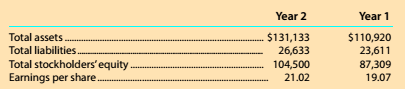Chapter 8, Problem 8.10.1MBA

Chapter
Section
Textbook Problem

Debt and price-earnings ratios Alphabet (formerly known as Google) (GOOG) is a technology company that offers users Internet search and e-mail services. Google also developed the Android operating system for use with cell phones and other mobile devices. The following data (in millions) were adapted from a recent financial statement of Alphabet.Compute the debt ratio for Years 1 and 2. Round to one decimal place.

To determine

Concept Introduction:

Debt Ratio:

Debt ratio is the relationship between the Total liabilities and Total Assets of a corporation. Debt ratio shows the part of assets financed by debts. It is calculated by dividing total liabilities by total assets. The formula of debt ratio is as follows:

Debt Ratio = Total LiabilitiesTotal Assets

To Calculate:

The Debt Ratios for year 1 and 2

Explanation

The Debt Ratios for year 1 and 2 are calculated as follows:

 \$ in Millions Year 1 Year 2 Total Liabilities (A)

Still sussing out bartleby?

Check out a sample textbook solution.

See a sample solution

The Solution to Your Study Problems

Bartleby provides explanations to thousands of textbook problems written by our experts, many with advanced degrees!

Get Started

Why is the journal called a book of original entry?

College Accounting (Book Only): A Career Approach

ALTERNATIVE DIVIDEND POLICIES In 2013, Keenan Company paid dividends totaling 3,600,000 on net income of 10.8 m...

Fundamentals of Financial Management, Concise Edition (with Thomson ONE - Business School Edition, 1 term (6 months) Printed Access Card) (MindTap Course List)

Provide an example of each.

Foundations of Business (MindTap Course List)